## Introduction

The aim of this project is to use PCA (Principal Component Analysis) algorithm for dimension reduction on the McDonald’s menu dataset.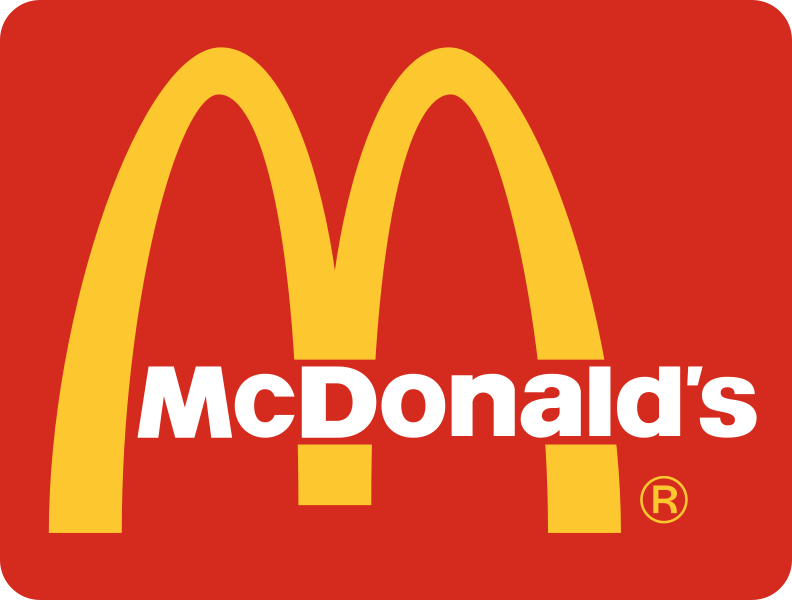Source: wikipedia.org

Problem of high dimensional data occurs when the dimension of the dataset (each numeric variable is a dimension) is large in comparison to number of observations. The goal of dimension reduction is to decrease the size of the dataset preserving as much information as possible.

## Dataset

Dataset used in this project contains nutritional values of 260 items from McDonald’s menu. Whole dataset can be found on kaggle website (https://www.kaggle.com/mcdonalds/nutrition-facts). Each item is described by 8 variables: saturated fat, cholesterol, sodium, carbohydrates, dietary fiber, sugars and protein.

#### Descriptive statistics:

summary(df) 
##  Saturated.Fat      Trans.Fat       Cholesterol         Sodium
##  Min.   : 0.000   Min.   :0.0000   Min.   :  0.00   Min.   :   0.0
##  1st Qu.: 1.000   1st Qu.:0.0000   1st Qu.:  5.00   1st Qu.: 107.5
##  Median : 5.000   Median :0.0000   Median : 35.00   Median : 190.0
##  Mean   : 6.008   Mean   :0.2038   Mean   : 54.94   Mean   : 495.8
##  3rd Qu.:10.000   3rd Qu.:0.0000   3rd Qu.: 65.00   3rd Qu.: 865.0
##  Max.   :20.000   Max.   :2.5000   Max.   :575.00   Max.   :3600.0
##  Carbohydrates    Dietary.Fiber       Sugars          Protein
##  Min.   :  0.00   Min.   :0.000   Min.   :  0.00   Min.   : 0.00
##  1st Qu.: 30.00   1st Qu.:0.000   1st Qu.:  5.75   1st Qu.: 4.00
##  Median : 44.00   Median :1.000   Median : 17.50   Median :12.00
##  Mean   : 47.35   Mean   :1.631   Mean   : 29.42   Mean   :13.34
##  3rd Qu.: 60.00   3rd Qu.:3.000   3rd Qu.: 48.00   3rd Qu.:19.00
##  Max.   :141.00   Max.   :7.000   Max.   :128.00   Max.   :87.00

#### Histograms:

hist.data.frame(df)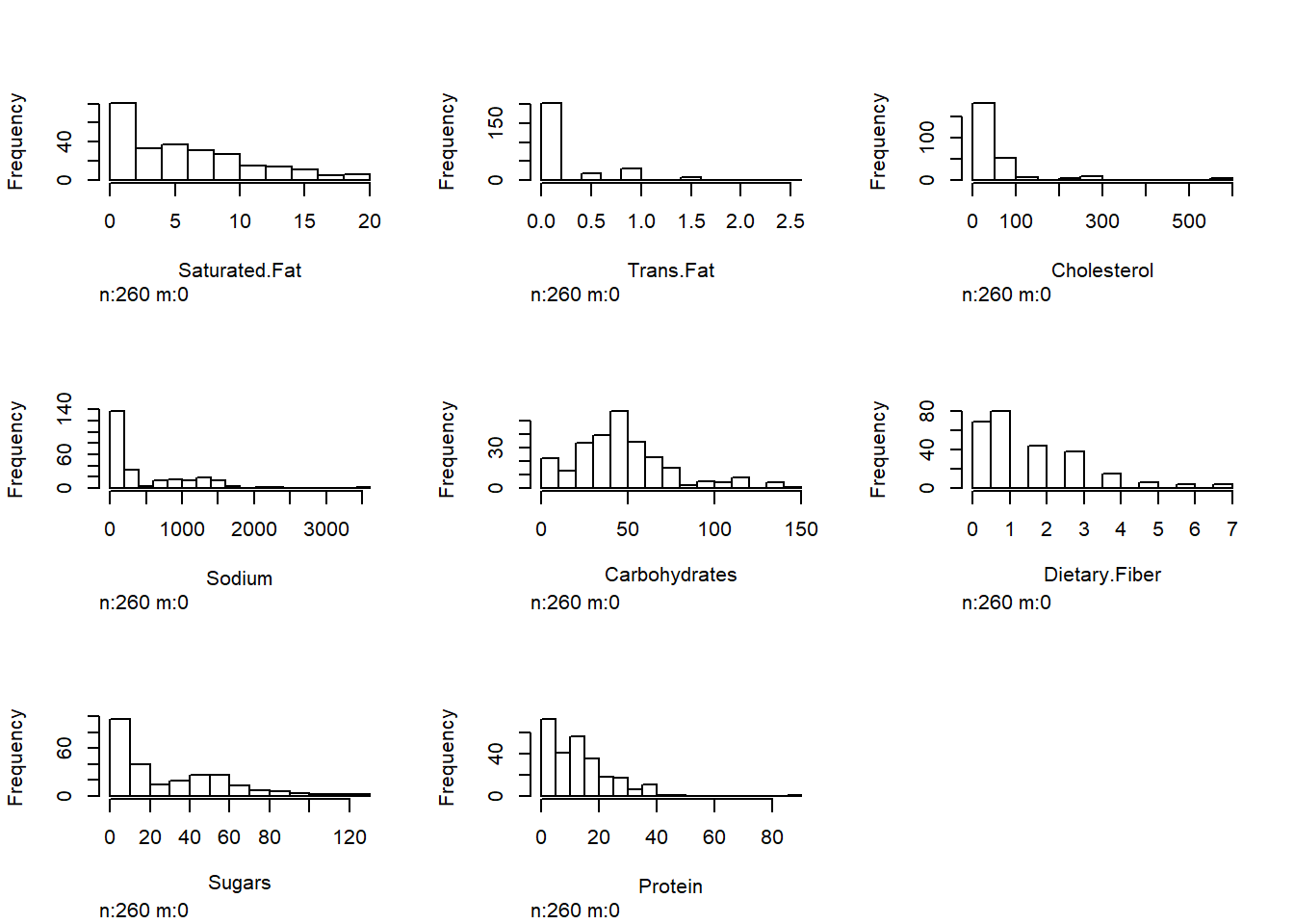#### Dimensions of the dataset:

dim(df)
##  260   8

Before further analysis the data has been normalized.

preproc <- preProcess(df, method=c("center", "scale"))
df_norm <- predict(preproc, df)

#### Matrix of plots:

ggpairs(df_norm)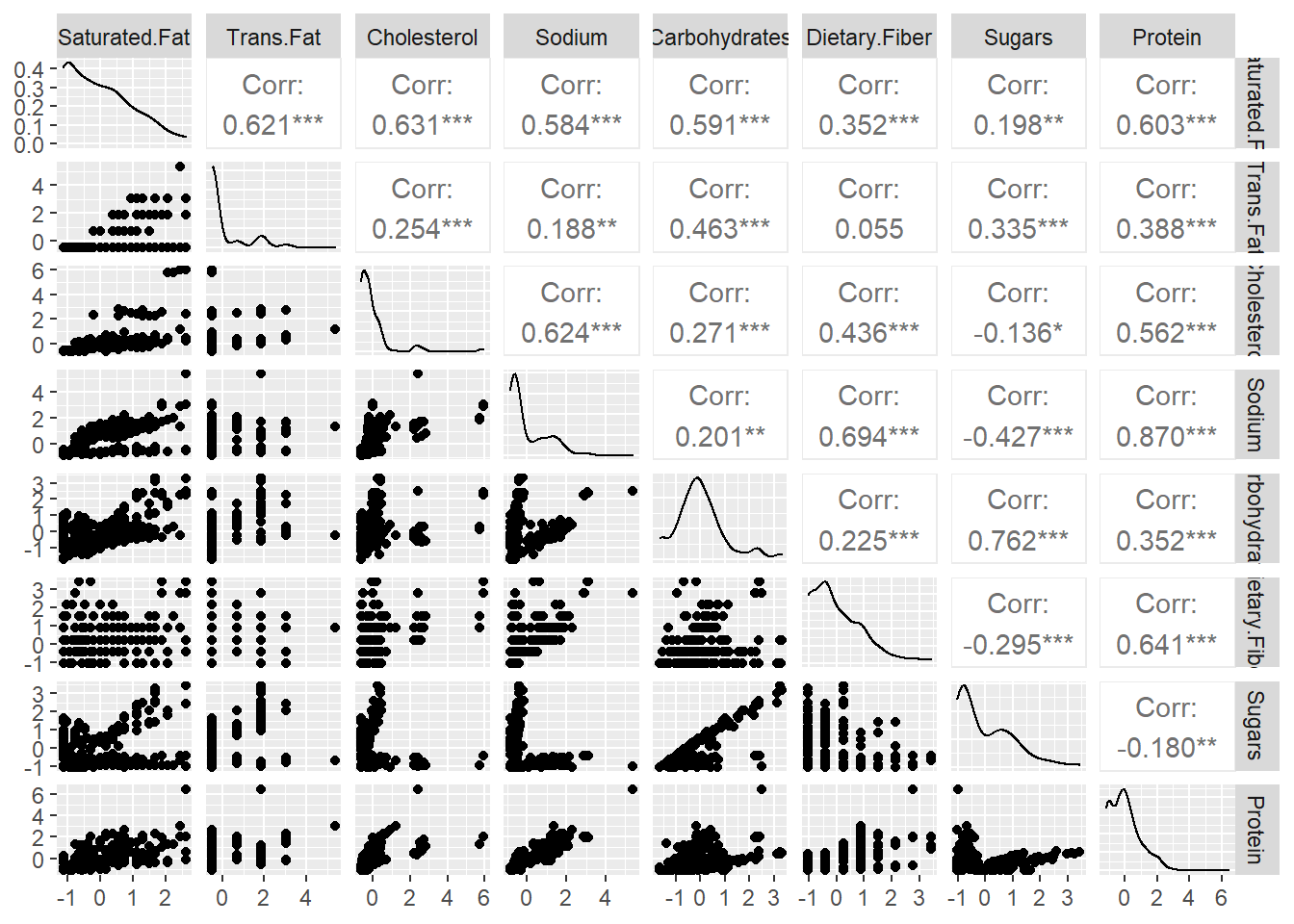#### Correlation matrix:

cor<-cor(df_norm, method="pearson")
corrplot(cor)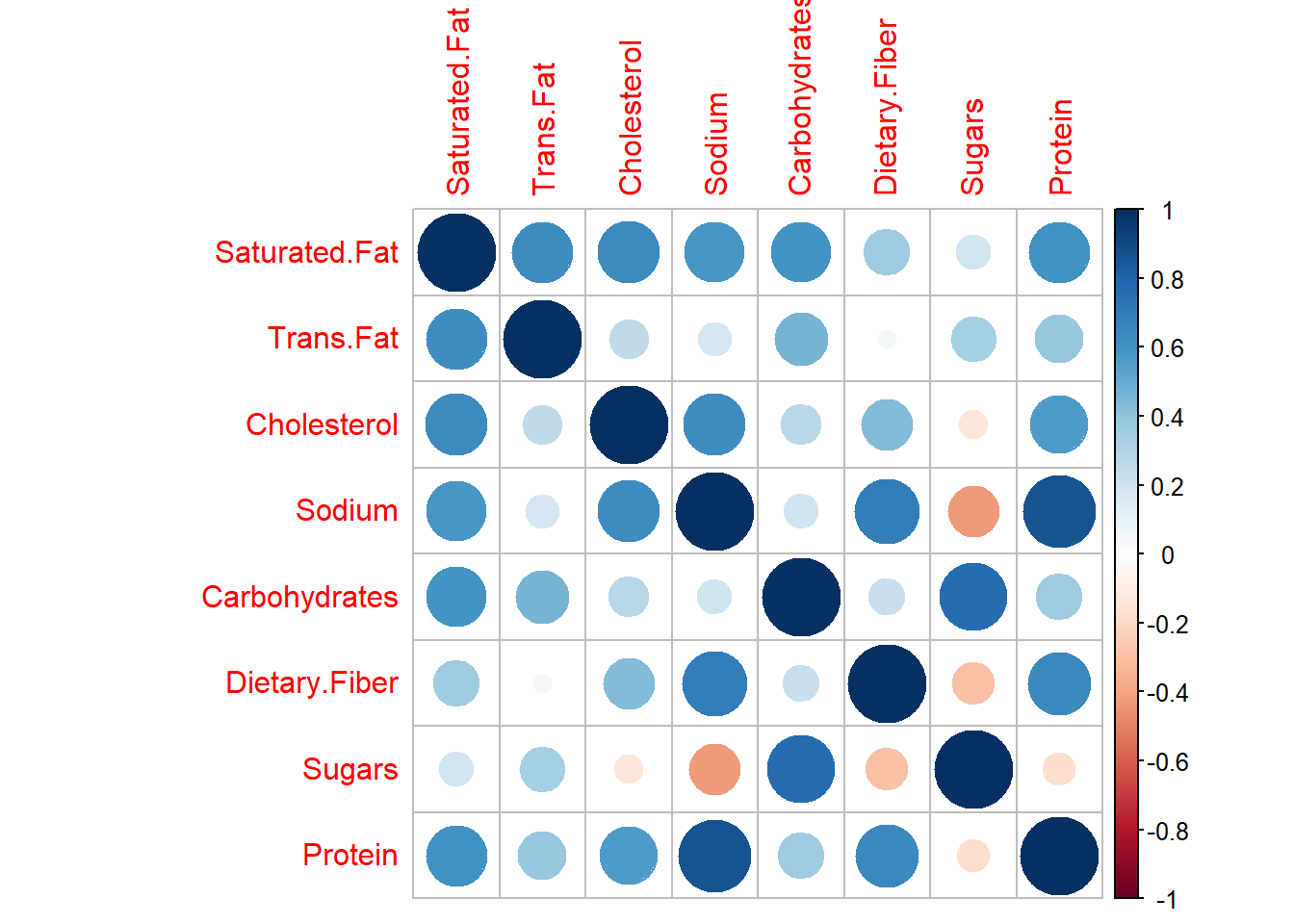On the correlation matrix it’s visible that some variables are positively correlated with saturated fat. Also the correlation between sugars and carbohydrates as well as protein and sodium can be easily spotted.

## PCA

pca <- prcomp(df_norm, center=FALSE, scale=FALSE)

PCA projections has been calculated using prcomp function which uses the singular value decomposition.

pca$rotation ## PC1 PC2 PC3 PC4 PC5 ## Saturated.Fat 0.43015581 -0.20009279 0.24851949 -0.16854817 0.02562347 ## Trans.Fat 0.26972412 -0.35490773 0.59813586 0.46222382 -0.35609428 ## Cholesterol 0.38495069 0.09019691 0.17808516 -0.77036509 -0.22396712 ## Sodium 0.43534374 0.29004074 -0.04388044 0.07805226 0.38904047 ## Carbohydrates 0.28112075 -0.49009859 -0.43369106 -0.03968195 0.12560935 ## Dietary.Fiber 0.34879197 0.26371707 -0.52107052 0.23873634 -0.67274191 ## Sugars -0.01137713 -0.64401117 -0.29283307 -0.08508928 0.05224139 ## Protein 0.45131975 0.13134138 -0.03844621 0.30426344 0.44714426 ## PC6 PC7 PC8 ## Saturated.Fat 0.754695383 -0.33459351 -0.0508323501 ## Trans.Fat -0.228437310 0.22112893 0.0445791009 ## Cholesterol -0.409813854 0.01980159 -0.0001305312 ## Sodium 0.120147811 0.53703482 0.5139456870 ## Carbohydrates -0.005284034 0.43172477 -0.5375127853 ## Dietary.Fiber 0.072357358 -0.11878811 0.0914333823 ## Sugars -0.160991017 -0.25891590 0.6296380445 ## Protein -0.405816175 -0.53180480 -0.1938155433 ### Choosing number of components There are 3 most common methods used to select the number of components: • Kaiser rule Kaiser rule focuses on component’s eigenvalues. An eigenvalue is an index that indicates how good a component is as a summary of the data (if an eigenvalue equals to 1, it means that the component contains the same amount of information as a single variable). This approach suggests that only components with eigenvalues higher than 1 should be chosen. df_norm.cov<-cov(df_norm) df_norm.eigen<-eigen(df_norm.cov) df_norm.eigen$values
##  3.84428921 2.19472808 0.74712560 0.56469152 0.31383510 0.22552415 0.08849565
##  0.02131069

Eigenvalues displayed above indicate that only 2 components should be chosen.

• Scree plot

The second approach relies on the scree plot. This plot visualizes the eigenvalues of the components in the ascending order. Scree plot approach suggests that the appropriate number of components is the number of bars preceding the bend of the line connecting eigenvalues.

fviz_eig(pca, choice='eigenvalue')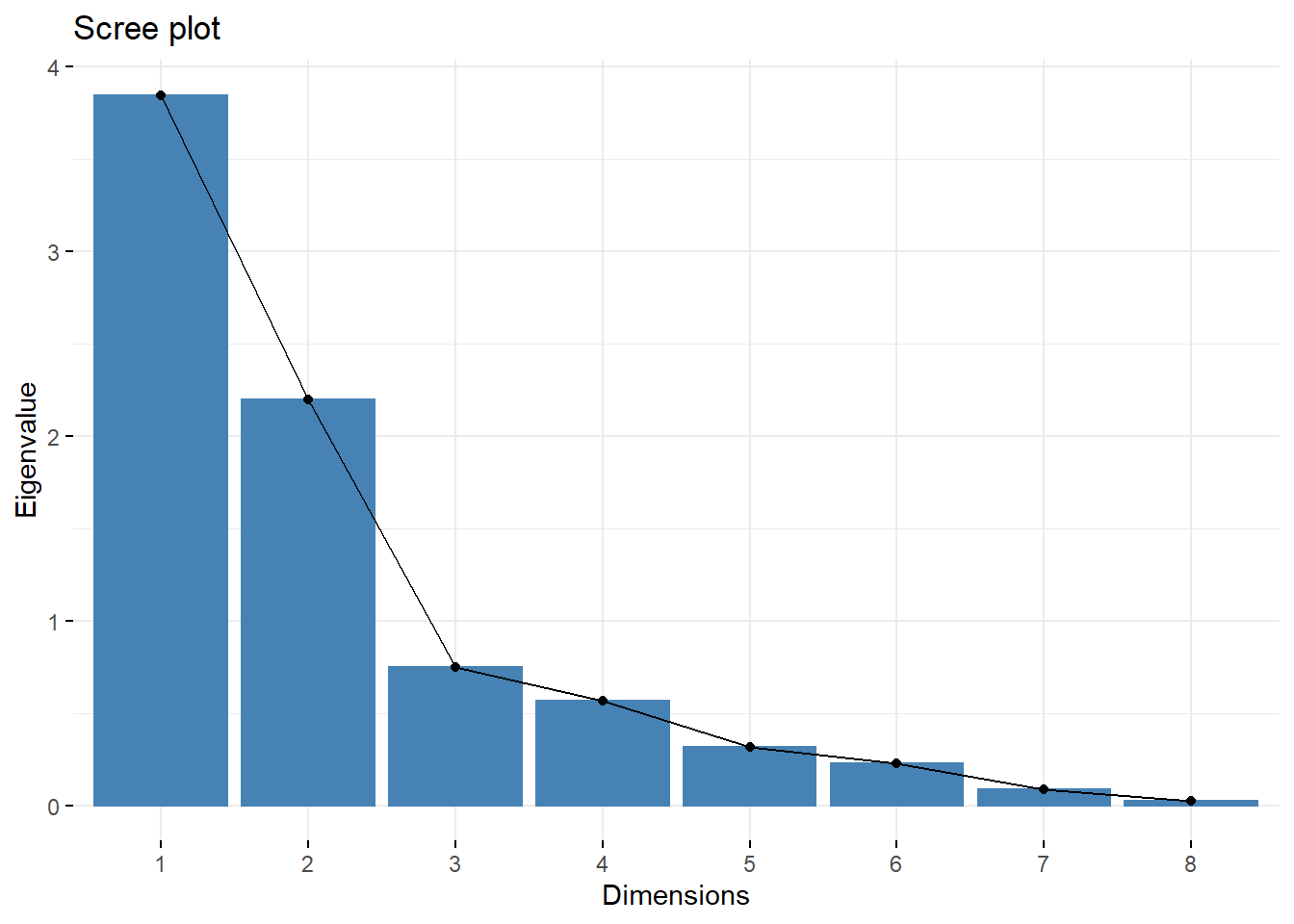This approach, as well as the Kaiser rule, indicates that the right number of components is 2.

• Proportion of variance explained

The last approach suggests that chosen components should explain over 2/3 of the variance.

summary(pca)
## Importance of components:
##                           PC1    PC2     PC3     PC4     PC5     PC6     PC7
## Standard deviation     1.9607 1.4815 0.86436 0.75146 0.56021 0.47489 0.29748
## Proportion of Variance 0.4805 0.2743 0.09339 0.07059 0.03923 0.02819 0.01106
## Cumulative Proportion  0.4805 0.7549 0.84827 0.91885 0.95808 0.98627 0.99734
##                            PC8
## Standard deviation     0.14598
## Proportion of Variance 0.00266
## Cumulative Proportion  1.00000
fviz_eig(pca)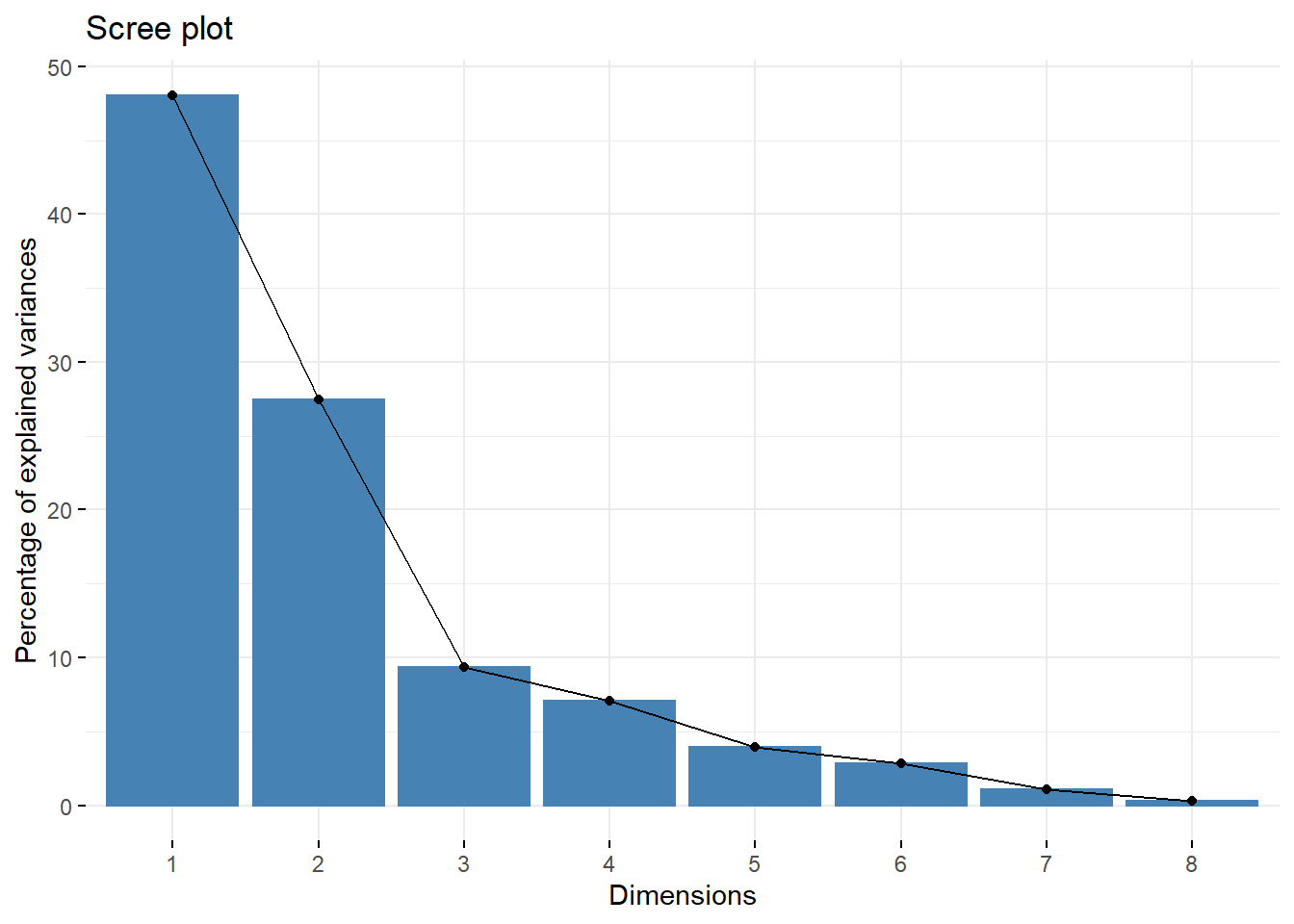Cumulative proportion of explained variance displayed above indicates that 4 components are able to explain over 90% of the variance. It means that this proportion of information can be preserved after reducing number of variables by half. First two components are able to explain over 3/4 of the variance so this number of components is enough. It means that results given by all three methods are the same.

### Components analysis

The “cloud of points” graph shows individual observations quality of representation.

fviz_pca_ind(pca, col.ind="cos2", geom = "point", gradient.cols = c("green", "yellow", "red" ))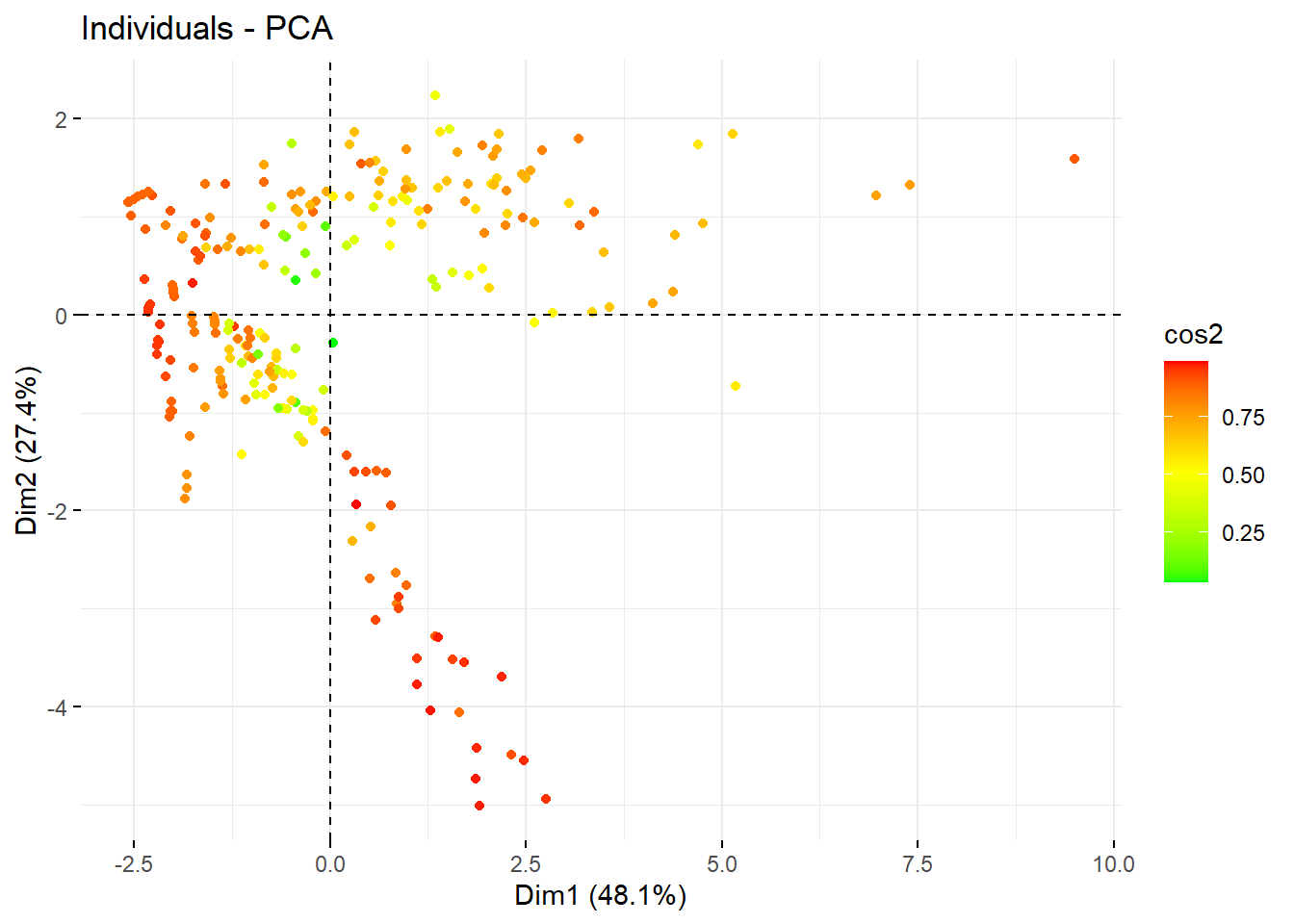fviz_pca_var(pca, col.var = "red")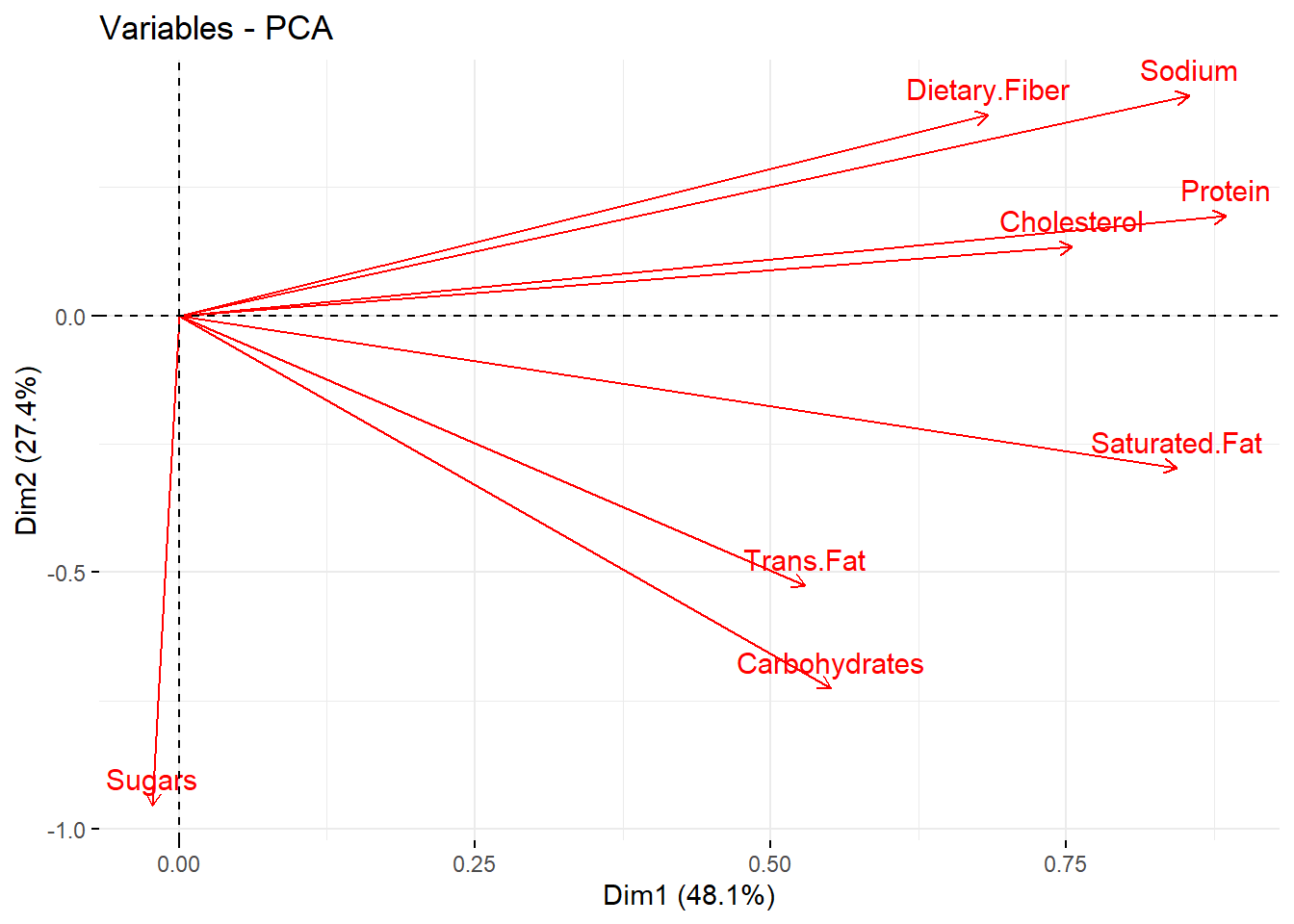The plot displayed above shows relations between variables as well as the “quality” of all factors. Variables correlated positively are close to each other whereas those correlated negatively are on the opposite sites of the plot. “Quality” of the variable is presented by the distance from the center - “the best” variables are protein and sodium. Just by looking on this graph, it’s hard to clearly distinguish the components.

Percentage of the contribution to first two components has been shown on plots displayed below.

PC1 <- fviz_contrib(pca, choice = "var", axes = 1)
PC2 <- fviz_contrib(pca, choice = "var", axes = 2)
grid.arrange(PC1, PC2)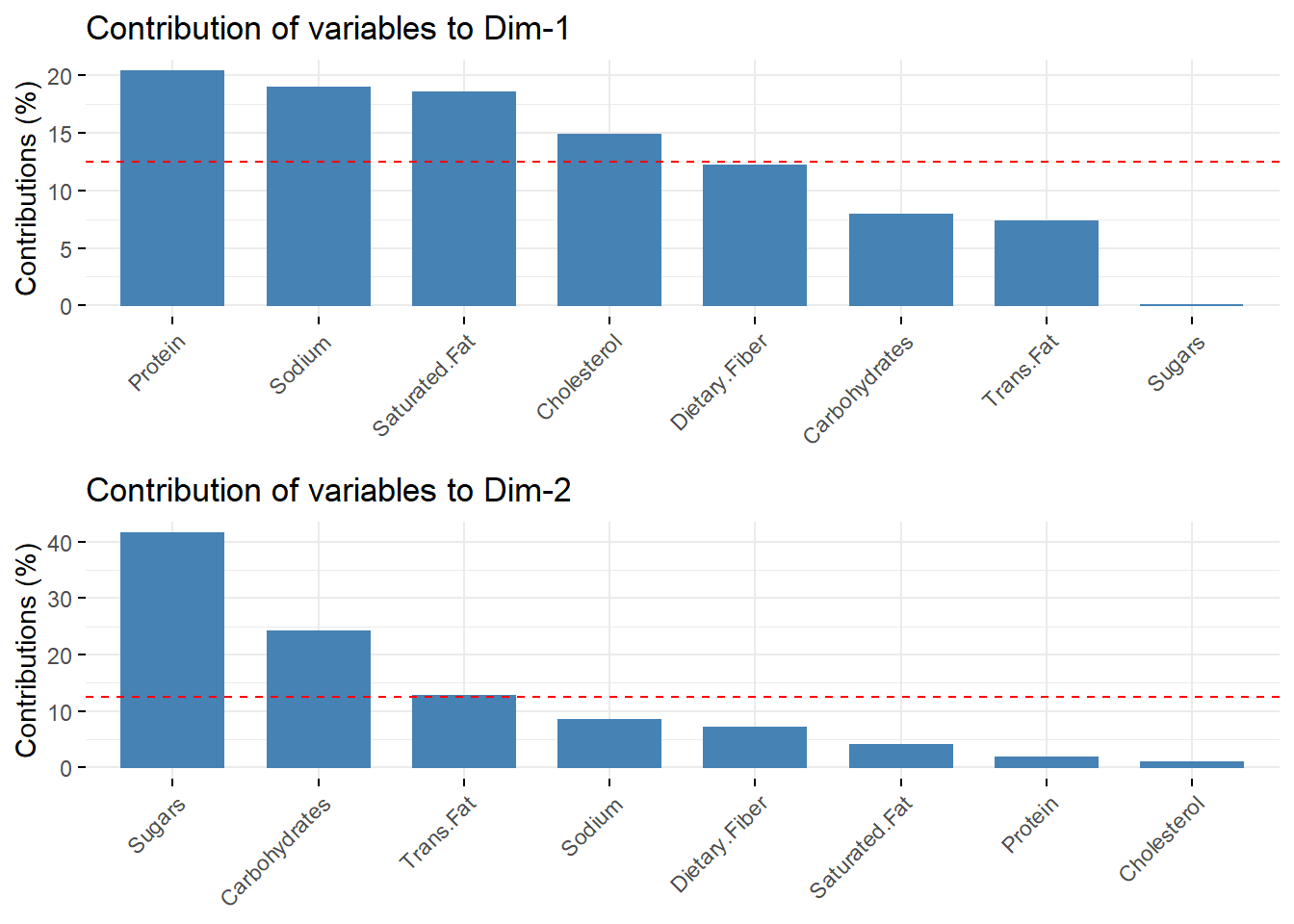On the first plot it’s visible that the first component consists of protein, sodium, saturated fat and cholesterol. The second one consists of sugars and carbohydrates.

## Conclusions

Dimension reduction simply refers to the process of reducing the number of dimensions in a dataset. The aim of this process is to preserve as much information as possible by reducing the number of features. Conducted research shows that over 90% of the variance can be explained by only a half of the variables and 2 variables out of 8 are able to keep over 3/4 of the information included in the original dataset. Dimension reduction techniques are very powerful when it comes to analysis and storage of huge datasets.